# Power Viewpoint

%matplotlib widget

from pacti import write_contracts_to_file
from pacti.contracts import PolyhedralIoContract
from pacti.iocontract import Var
from contract_utils import *
from plot_utils import plot_guarantees_with_bounds_hover


## Power viewpoint modeling¶

Objective: charge the spacecraft battery

As summarized in the qualitative impacts table, this function affects this viewpoint with impacts that are linear with the duration of the task: - the battery charges proportionally to a generation rate.

# Parameters:
# - s: index of the timeline variables
# - generation: (min, max) rate of battery charge during the task instance
def CHRG_power(s: int, generation: Tuple[float, float]) -> PolyhedralIoContract:
spec = PolyhedralIoContract.from_strings(
input_vars = [
f"soc{s}_entry",          # initial battery SOC
],
output_vars = [
f"soc{s}_exit",           # final battery SOC
],
assumptions = [
# Task has a positive scheduled duration
f"0 <= duration_charging{s}",
# Lower and upper bound on entry soc
f"0 <= soc{s}_entry <= 100.0",
# The increase under the maximum generation rate should not overcharge the battery.
f"soc{s}_entry + {generation}*duration_charging{s} <= 100",
],
guarantees = [
# duration*generation(min) <= soc{exit} - soc{entry} <= duration*generation(max)
f"{generation}*duration_charging{s} <= soc{s}_exit - soc{s}_entry <= {generation}*duration_charging{s}",

# Battery cannot exceed maximum SOC
f"soc{s}_exit <= 100.0",

# Battery should not completely discharge
f"0 <= soc{s}_exit",
])
return spec

charging1_power = CHRG_power(s=2, generation=(4.0, 5.0))
print(f"Contract charging1_power:\n\n{charging1_power}")

_ = plot_guarantees_with_bounds_hover(contract=charging1_power,
x_var=Var("duration_charging2"),
y_var=Var("soc2_exit"),
var_values={
Var("soc2_entry"):42,
},
x_lims=(0,100),
y_lims=(0,100))

Contract charging1_power:

InVars: [soc2_entry, duration_charging2]
OutVars:[soc2_exit]
A: [
-duration_charging2 <= -0
-soc2_entry <= -0
soc2_entry <= 100
5 duration_charging2 + soc2_entry <= 100
]
G: [
4 duration_charging2 + soc2_entry - soc2_exit <= 0
-5 duration_charging2 - soc2_entry + soc2_exit <= 0
]

Figure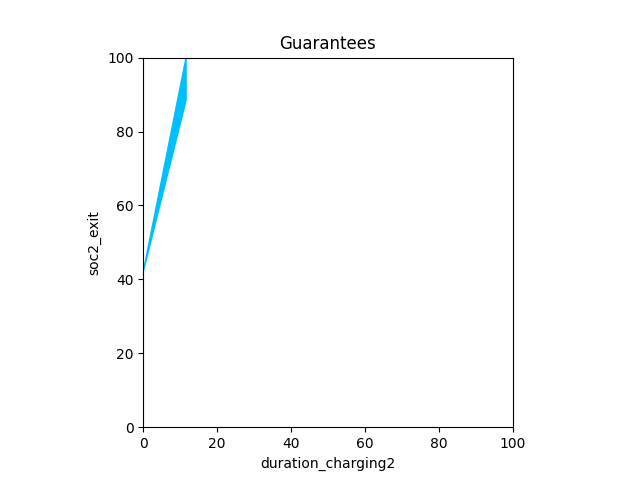### Power-consuming Tasks (DSN, SBO, TCM)¶

# Parameters:
# - s: start index of the timeline variables
# - consumption: (min, max) rate of battery discharge during the task instance
def power_consumer(s: int, task: str, consumption: Tuple[float, float]) -> PolyhedralIoContract:
spec = PolyhedralIoContract.from_strings(
input_vars = [
f"soc{s}_entry",          # initial battery SOC
],
output_vars = [
f"soc{s}_exit",           # final battery SOC
],
assumptions = [
# Task has a positive scheduled duration
# Upper bound on entry soc
f"soc{s}_entry <= 100.0",
# Lower bound on entry soc
f"0 <= soc{s}_entry",
# Battery has enough energy for worst-case consumption throughout the task instance
],
guarantees=[
# duration*consumption(min) <= soc{entry} - soc{exit} <= duration*consumption(max)
# Battery cannot exceed maximum SOC
f"soc{s}_exit <= 100",
# Battery should not completely discharge
f"0 <= soc{s}_exit",
])
return spec


Objective: downlink science data to Earth.

As summarized in the qualitative impacts table, this function affects this viewpoint with impacts that are linear with the duration of the task: - the battery discharges proportionally to a consumption rate.

dsn1_power = power_consumer(s=1, task="dsn", consumption=(2.0, 2.2))
print(f"Contract dsn1_power:\n\n{dsn1_power}")

_ = plot_guarantees_with_bounds_hover(contract=dsn1_power,
x_var=Var("duration_dsn1"),
y_var=Var("soc1_exit"),
var_values={
Var("soc1_entry"):80,
},
x_lims=(0,20),
y_lims=(0,80))

Contract dsn1_power:

InVars: [soc1_entry, duration_dsn1]
OutVars:[soc1_exit]
A: [
-duration_dsn1 <= -0
soc1_entry <= 100
-soc1_entry <= -0
2.2 duration_dsn1 - soc1_entry <= 0
]
G: [
2 duration_dsn1 - soc1_entry + soc1_exit <= 0
-2.2 duration_dsn1 + soc1_entry - soc1_exit <= 0
]

Figure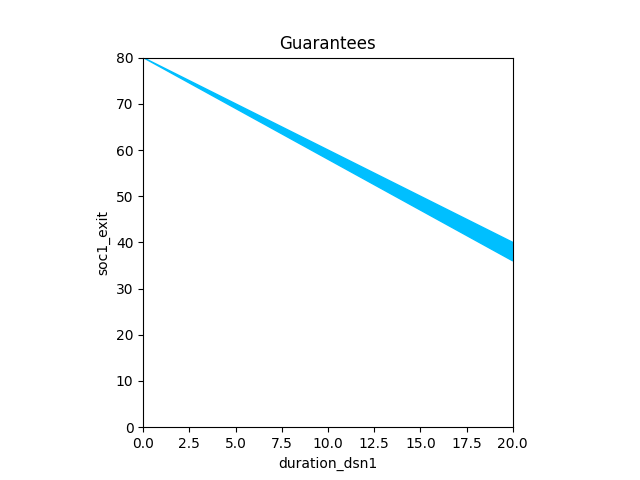### SBO Task (Small body observations)¶

Objective: Acquire small body observations (science data & navigation)

As summarized in the qualitative impacts table, this function affects this viewpoint with impacts that are linear with the duration of the task: - the battery discharges proportionally to a consumption rate.

sbo1_power = power_consumer(s=3, task="sbo", consumption=(0.1, 0.2))
print(f"Contract sbo1_power:\n\n{sbo1_power}")

_ = plot_guarantees_with_bounds_hover(contract=sbo1_power,
x_var=Var("duration_sbo3"),
y_var=Var("soc3_exit"),
var_values={
Var("soc3_entry"):80,
},
x_lims=(0,100),
y_lims=(0,100))

Contract sbo1_power:

InVars: [soc3_entry, duration_sbo3]
OutVars:[soc3_exit]
A: [
-duration_sbo3 <= -0
soc3_entry <= 100
-soc3_entry <= -0
0.2 duration_sbo3 - soc3_entry <= 0
]
G: [
0.1 duration_sbo3 - soc3_entry + soc3_exit <= 0
-0.2 duration_sbo3 + soc3_entry - soc3_exit <= 0
]

Figure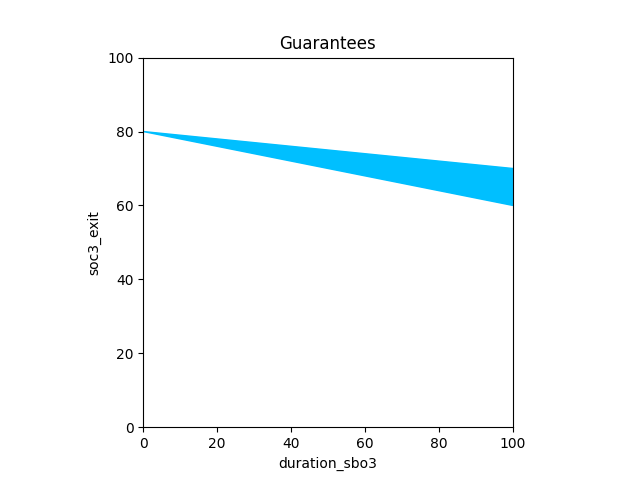### TCM Task (Perform a Trajectory Correction Maneuver)¶

Objective: Perform a delta-V maneuver to bring the spacecraft trajectory closer to that of the small body.

As summarized in the qualitative impacts table, this function affects this viewpoint with impacts that are linear with the duration of the task: - Power: the thrusters must be heated before firing them, thereby discharging the battery proportionally to a consumption rate.

Since heating the thruster must happen just before firing them, this task is modeled as the composition of two subtasks: Heating and DeltaV.

tcm1_heating_power = power_consumer(s=4, task="tcm_heating", consumption=(0.7, 0.8))
print(f"Contract tcm1_heating_power:\n\n{tcm1_heating_power}")

_ = plot_guarantees_with_bounds_hover(contract=tcm1_heating_power,
x_var=Var("duration_tcm_heating4"),
y_var=Var("soc4_exit"),
var_values={
Var("soc4_entry"):80,
},
x_lims=(0,100),
y_lims=(0,100))

Contract tcm1_heating_power:

InVars: [soc4_entry, duration_tcm_heating4]
OutVars:[soc4_exit]
A: [
-duration_tcm_heating4 <= -0
soc4_entry <= 100
-soc4_entry <= -0
0.8 duration_tcm_heating4 - soc4_entry <= 0
]
G: [
0.7 duration_tcm_heating4 - soc4_entry + soc4_exit <= 0
-0.8 duration_tcm_heating4 + soc4_entry - soc4_exit <= 0
]

Figure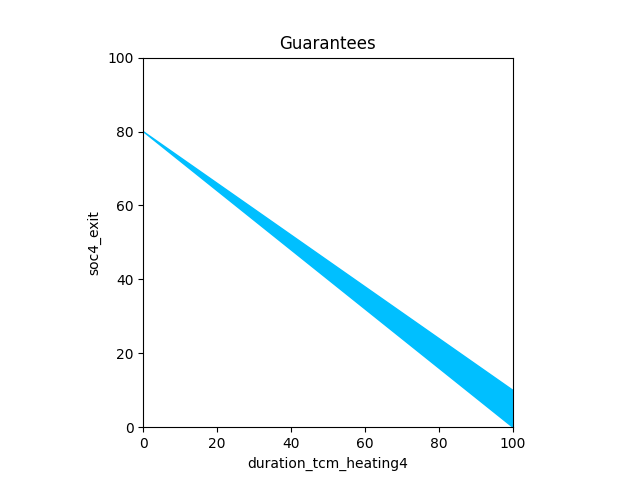tcm1_deltav_power = power_consumer(s=5, task="tcm_deltav", consumption=(0.2, 0.3))
print(f"Contract tcm1_deltav_power:\n\n{tcm1_deltav_power}")

_ = plot_guarantees_with_bounds_hover(contract=tcm1_deltav_power,
x_var=Var("duration_tcm_deltav5"),
y_var=Var("soc5_exit"),
var_values={
Var("soc5_entry"):80,
},
x_lims=(0,100),
y_lims=(0,100))

Contract tcm1_deltav_power:

InVars: [soc5_entry, duration_tcm_deltav5]
OutVars:[soc5_exit]
A: [
-duration_tcm_deltav5 <= -0
soc5_entry <= 100
-soc5_entry <= -0
0.3 duration_tcm_deltav5 - soc5_entry <= 0
]
G: [
0.2 duration_tcm_deltav5 - soc5_entry + soc5_exit <= 0
-0.3 duration_tcm_deltav5 + soc5_entry - soc5_exit <= 0
]

Figure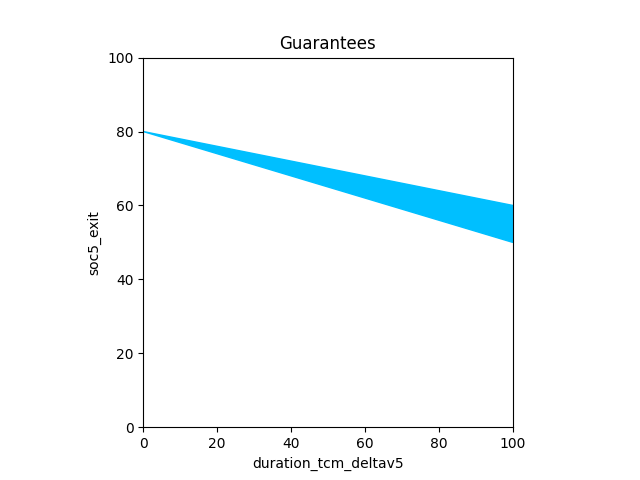Algebraic composition allows us to capture the requirement that a TCM Heating subtask must always precede a TCM DeltaV subtask by composing them.

tcm1_power=scenario_sequence(c1=tcm1_heating_power, c2=tcm1_deltav_power, variables=["soc"], c1index=4)
print(f"========= tcm1_power\n{tcm1_power}")
print('\n'.join(bounds(tcm1_power)))

========= tcm1_power
InVars: [soc4_entry, duration_tcm_heating4, duration_tcm_deltav5]
OutVars:[soc5_exit, output_soc4]
A: [
-duration_tcm_deltav5 <= 0
0.3 duration_tcm_deltav5 + 0.8 duration_tcm_heating4 - soc4_entry <= 0
-duration_tcm_heating4 <= 0
soc4_entry <= 100
]
G: [
0.7 duration_tcm_heating4 + output_soc4 - soc4_entry <= 0
-0.8 duration_tcm_heating4 - output_soc4 + soc4_entry <= 0
0.2 duration_tcm_deltav5 - output_soc4 + soc5_exit <= 0
-0.3 duration_tcm_deltav5 + output_soc4 - soc5_exit <= 0
]
input duration_tcm_deltav5 in [0.00,333.33]
input duration_tcm_heating4 in [0.00,125.00]
input soc4_entry in [0.00,100.00]
output output_soc4 in [0.00,100.00]
output soc5_exit in [-0.00,100.00]


### Power Schedule Analysis¶

Let's consider a simple 4-step schedule of the following sequence of task instances, which we compose: - DSN - CHARGING - SBO - TCM

steps12=scenario_sequence(c1=dsn1_power, c2=charging1_power, variables=["soc"], c1index=1)
print(f"---- L2R Steps 1,2\n{steps12}")
print('\n'.join(bounds(steps12)))

---- L2R Steps 1,2
InVars: [soc1_entry, duration_dsn1, duration_charging2]
OutVars:[soc2_exit, output_soc1]
A: [
-duration_charging2 <= 0
5 duration_charging2 - 2 duration_dsn1 + soc1_entry <= 100
-duration_dsn1 <= 0
soc1_entry <= 100
2.2 duration_dsn1 - soc1_entry <= 0
]
G: [
2 duration_dsn1 + output_soc1 - soc1_entry <= 0
-2.2 duration_dsn1 - output_soc1 + soc1_entry <= 0
4 duration_charging2 + output_soc1 - soc2_exit <= 0
-5 duration_charging2 - output_soc1 + soc2_exit <= 0
]
input duration_charging2 in [0.00,20.00]
input duration_dsn1 in [0.00,45.45]
input soc1_entry in [0.00,100.00]
output output_soc1 in [0.00,100.00]
output soc2_exit in [0.00,100.00]

steps23=scenario_sequence(c1=charging1_power, c2=sbo1_power, variables=["soc"], c1index=2)
print(f"---- L2R Steps 2,3\n{steps23}")
print('\n'.join(bounds(steps23)))

---- L2R Steps 2,3
InVars: [soc2_entry, duration_charging2, duration_sbo3]
OutVars:[soc3_exit, output_soc2]
A: [
-duration_sbo3 <= 0
-4 duration_charging2 + 0.2 duration_sbo3 - soc2_entry <= 0
-duration_charging2 <= 0
-soc2_entry <= 0
5 duration_charging2 + soc2_entry <= 100
]
G: [
4 duration_charging2 - output_soc2 + soc2_entry <= 0
-5 duration_charging2 + output_soc2 - soc2_entry <= 0
0.1 duration_sbo3 - output_soc2 + soc3_exit <= 0
-0.2 duration_sbo3 + output_soc2 - soc3_exit <= 0
]
input duration_charging2 in [0.00,20.00]
input duration_sbo3 in [0.00,500.00]
input soc2_entry in [0.00,100.00]
output output_soc2 in [0.00,100.00]
output soc3_exit in [0.00,100.00]

steps1_23=scenario_sequence(c1=dsn1_power, c2=steps23, variables=["soc"], c1index=1)
print(f"---- L2R Steps 1 and 2,3\n{steps1_23}")
print('\n'.join(bounds(steps1_23)))

---- L2R Steps 1 and 2,3
InVars: [soc1_entry, duration_dsn1, duration_charging2, duration_sbo3]
OutVars:[soc3_exit, output_soc2, output_soc1]
A: [
-duration_sbo3 <= 0
-4 duration_charging2 + 2.2 duration_dsn1 + 0.2 duration_sbo3 - soc1_entry <= 0
-duration_charging2 <= 0
5 duration_charging2 - 2 duration_dsn1 + soc1_entry <= 100
-duration_dsn1 <= 0
soc1_entry <= 100
2.2 duration_dsn1 - soc1_entry <= 0
]
G: [
2 duration_dsn1 + output_soc1 - soc1_entry <= 0
-2.2 duration_dsn1 - output_soc1 + soc1_entry <= 0
4 duration_charging2 + output_soc1 - output_soc2 <= 0
-5 duration_charging2 - output_soc1 + output_soc2 <= 0
0.1 duration_sbo3 - output_soc2 + soc3_exit <= 0
-0.2 duration_sbo3 + output_soc2 - soc3_exit <= 0
]
input duration_charging2 in [0.00,20.00]
input duration_dsn1 in [0.00,45.45]
input duration_sbo3 in [0.00,500.00]
input soc1_entry in [0.00,100.00]
output output_soc1 in [0.00,100.00]
output output_soc2 in [0.00,100.00]
output soc3_exit in [0.00,100.00]

steps123=scenario_sequence(c1=steps12, c2=sbo1_power, variables=["soc"], c1index=2)
print(f"---- L2R Steps 1,2,3\n{steps123}")
print('\n'.join(bounds(steps123)))

---- L2R Steps 1,2,3
InVars: [soc1_entry, duration_dsn1, duration_charging2, duration_sbo3]
OutVars:[output_soc1, soc3_exit, output_soc2]
A: [
-duration_sbo3 <= 0
-4 duration_charging2 + 2 duration_dsn1 + 0.2 duration_sbo3 - soc1_entry <= 0
-duration_charging2 <= 0
5 duration_charging2 - 2 duration_dsn1 + soc1_entry <= 100
-duration_dsn1 <= 0
soc1_entry <= 100
2.2 duration_dsn1 - soc1_entry <= 0
]
G: [
2 duration_dsn1 + output_soc1 - soc1_entry <= 0
-2.2 duration_dsn1 - output_soc1 + soc1_entry <= 0
4 duration_charging2 + output_soc1 - output_soc2 <= 0
-5 duration_charging2 - output_soc1 + output_soc2 <= 0
0.1 duration_sbo3 - output_soc2 + soc3_exit <= 0
-0.2 duration_sbo3 + output_soc2 - soc3_exit <= 0
]
input duration_charging2 in [0.00,20.00]
input duration_dsn1 in [0.00,45.45]
input duration_sbo3 in [0.00,500.00]
input soc1_entry in [0.00,100.00]
output output_soc1 in [0.00,100.00]
output output_soc2 in [0.00,100.00]
output soc3_exit in [-9.09,100.00]

back34=scenario_sequence(c1=sbo1_power, c2=tcm1_power, variables=["soc"], c1index=3)
print(f"---- R2L Steps 3,4\n{back34}")
print('\n'.join(bounds(back34)))

---- R2L Steps 3,4
InVars: [soc3_entry, duration_sbo3, duration_tcm_heating4, duration_tcm_deltav5]
OutVars:[soc5_exit, output_soc4, output_soc3]
A: [
-duration_tcm_deltav5 <= 0
0.2 duration_sbo3 + 0.3 duration_tcm_deltav5 + 0.8 duration_tcm_heating4 - soc3_entry <= 0
-duration_tcm_heating4 <= 0
-duration_sbo3 <= 0
soc3_entry <= 100
]
G: [
0.1 duration_sbo3 + output_soc3 - soc3_entry <= 0
-0.2 duration_sbo3 - output_soc3 + soc3_entry <= 0
0.7 duration_tcm_heating4 - output_soc3 + output_soc4 <= 0
-0.8 duration_tcm_heating4 + output_soc3 - output_soc4 <= 0
0.2 duration_tcm_deltav5 - output_soc4 + soc5_exit <= 0
-0.3 duration_tcm_deltav5 + output_soc4 - soc5_exit <= 0
]
input duration_sbo3 in [0.00,500.00]
input duration_tcm_deltav5 in [0.00,333.33]
input duration_tcm_heating4 in [0.00,125.00]
input soc3_entry in [0.00,100.00]
output output_soc3 in [0.00,100.00]
output output_soc4 in [0.00,100.00]
output soc5_exit in [-0.00,100.00]

back234=scenario_sequence(c1=charging1_power, c2=back34, variables=["soc"], c1index=2)
print(f"---- R2L Steps 2,3,4\n{back234}")
print('\n'.join(bounds(back234)))

---- R2L Steps 2,3,4
InVars: [soc2_entry, duration_charging2, duration_sbo3, duration_tcm_heating4, duration_tcm_deltav5]
OutVars:[soc5_exit, output_soc4, output_soc3, output_soc2]
A: [
-duration_tcm_deltav5 <= 0
-4 duration_charging2 + 0.2 duration_sbo3 + 0.3 duration_tcm_deltav5 + 0.8 duration_tcm_heating4 - soc2_entry <= 0
-duration_tcm_heating4 <= 0
-duration_sbo3 <= 0
-duration_charging2 <= 0
-soc2_entry <= 0
5 duration_charging2 + soc2_entry <= 100
]
G: [
4 duration_charging2 - output_soc2 + soc2_entry <= 0
-5 duration_charging2 + output_soc2 - soc2_entry <= 0
0.1 duration_sbo3 - output_soc2 + output_soc3 <= 0
-0.2 duration_sbo3 + output_soc2 - output_soc3 <= 0
0.7 duration_tcm_heating4 - output_soc3 + output_soc4 <= 0
-0.8 duration_tcm_heating4 + output_soc3 - output_soc4 <= 0
0.2 duration_tcm_deltav5 - output_soc4 + soc5_exit <= 0
-0.3 duration_tcm_deltav5 + output_soc4 - soc5_exit <= 0
]
input duration_charging2 in [0.00,20.00]
input duration_sbo3 in [0.00,500.00]
input duration_tcm_deltav5 in [0.00,333.33]
input duration_tcm_heating4 in [0.00,125.00]
input soc2_entry in [0.00,100.00]
output output_soc2 in [0.00,100.00]
output output_soc3 in [0.00,100.00]
output output_soc4 in [0.00,100.00]
output soc5_exit in [0.00,100.00]

back1234=scenario_sequence(c1=dsn1_power, c2=back234, variables=["soc"], c1index=1).rename_variables([("soc5_exit", "output_soc5")])
print(f"---- Steps 1,2,3,4 R2L\n{back1234}")
print('\n'.join(bounds(back1234)))

---- Steps 1,2,3,4 R2L
InVars: [soc1_entry, duration_dsn1, duration_charging2, duration_sbo3, duration_tcm_heating4, duration_tcm_deltav5]
OutVars:[output_soc4, output_soc3, output_soc2, output_soc1, output_soc5]
A: [
-duration_tcm_deltav5 <= 0
-4 duration_charging2 + 2.2 duration_dsn1 + 0.2 duration_sbo3 + 0.3 duration_tcm_deltav5 + 0.8 duration_tcm_heating4 - soc1_entry <= 0
-duration_tcm_heating4 <= 0
-duration_sbo3 <= 0
-duration_charging2 <= 0
5 duration_charging2 - 2 duration_dsn1 + soc1_entry <= 100
-duration_dsn1 <= 0
soc1_entry <= 100
2.2 duration_dsn1 - soc1_entry <= 0
]
G: [
2 duration_dsn1 + output_soc1 - soc1_entry <= 0
-2.2 duration_dsn1 - output_soc1 + soc1_entry <= 0
4 duration_charging2 + output_soc1 - output_soc2 <= 0
-5 duration_charging2 - output_soc1 + output_soc2 <= 0
0.1 duration_sbo3 - output_soc2 + output_soc3 <= 0
-0.2 duration_sbo3 + output_soc2 - output_soc3 <= 0
0.7 duration_tcm_heating4 - output_soc3 + output_soc4 <= 0
-0.8 duration_tcm_heating4 + output_soc3 - output_soc4 <= 0
0.2 duration_tcm_deltav5 - output_soc4 + output_soc5 <= 0
-0.3 duration_tcm_deltav5 + output_soc4 - output_soc5 <= 0
]
input duration_charging2 in [0.00,20.00]
input duration_dsn1 in [0.00,45.45]
input duration_sbo3 in [0.00,500.00]
input duration_tcm_deltav5 in [0.00,333.33]
input duration_tcm_heating4 in [0.00,125.00]
input soc1_entry in [0.00,100.00]
output output_soc1 in [0.00,100.00]
output output_soc2 in [0.00,100.00]
output output_soc3 in [0.00,100.00]
output output_soc4 in [0.00,100.00]
output output_soc5 in [0.00,100.00]

steps1234=scenario_sequence(c1=steps123, c2=tcm1_power, variables=["soc"], c1index=3).rename_variables([("soc5_exit", "output_soc5")])
print(f"---- Steps 1,2,3,4 L2R\n{steps1234}")
print('\n'.join(bounds(steps1234)))

---- Steps 1,2,3,4 L2R
InVars: [soc1_entry, duration_dsn1, duration_charging2, duration_sbo3, duration_tcm_heating4, duration_tcm_deltav5]
OutVars:[output_soc1, output_soc2, output_soc4, output_soc3, output_soc5]
A: [
-duration_tcm_deltav5 <= 0
-5 duration_charging2 + 2 duration_dsn1 + 0.2 duration_sbo3 + 0.3 duration_tcm_deltav5 + 0.8 duration_tcm_heating4 - soc1_entry <= 0
-duration_tcm_heating4 <= 0
-duration_sbo3 <= 0
-4 duration_charging2 + 2 duration_dsn1 + 0.2 duration_sbo3 - soc1_entry <= 0
-duration_charging2 <= 0
5 duration_charging2 - 2 duration_dsn1 + soc1_entry <= 100
-duration_dsn1 <= 0
soc1_entry <= 100
2.2 duration_dsn1 - soc1_entry <= 0
]
G: [
2 duration_dsn1 + output_soc1 - soc1_entry <= 0
-2.2 duration_dsn1 - output_soc1 + soc1_entry <= 0
4 duration_charging2 + output_soc1 - output_soc2 <= 0
-5 duration_charging2 - output_soc1 + output_soc2 <= 0
0.1 duration_sbo3 - output_soc2 + output_soc3 <= 0
-0.2 duration_sbo3 + output_soc2 - output_soc3 <= 0
0.7 duration_tcm_heating4 - output_soc3 + output_soc4 <= 0
-0.8 duration_tcm_heating4 + output_soc3 - output_soc4 <= 0
0.2 duration_tcm_deltav5 - output_soc4 + output_soc5 <= 0
-0.3 duration_tcm_deltav5 + output_soc4 - output_soc5 <= 0
]
input duration_charging2 in [0.00,20.00]
input duration_dsn1 in [0.00,45.45]
input duration_sbo3 in [0.00,500.00]
input duration_tcm_deltav5 in [0.00,333.33]
input duration_tcm_heating4 in [0.00,125.00]
input soc1_entry in [0.00,100.00]
output output_soc1 in [0.00,100.00]
output output_soc2 in [0.00,100.00]
output output_soc3 in [-9.09,100.00]
output output_soc4 in [-27.27,100.00]
output output_soc5 in [-27.27,100.00]

print(f"L2R refines R2L? : {steps1234.refines(back1234)}")
print(f"R2L refines L2R? : {back1234.refines(steps1234)}")

L2R refines R2L? : True
R2L refines L2R? : False


#### Power Schedule constraints¶

write_contracts_to_file(
contracts=[steps1234, back1234],
names=["scenario_power_left_to_right_composition", "scenario_power_right_to_left_composition"],
file_name="json/scenario_power.json")